# Seaborn | Regression Plots

The regression plots in seaborn are primarily intended to add a visual guide that helps to emphasize patterns in a dataset during exploratory data analyses. Regression plots as the name suggests creates a regression line between 2 parameters and helps to visualize their linear relationships. This article deals with those kinds of plots in seaborn and shows the ways that can be adapted to change the size, aspect, ratio etc. of such plots.

Seaborn is not only a visualization library but also a provider of built-in datasets. Here, we will be working with one of such datasets in seaborn named ‘tips’. The tips dataset contains information about the people who probably had food at the restaurant and whether or not they left a tip. It also provides information about the gender of the people, whether they smoke, day, time and so on.

Let us have a look at the dataset first before we start with the regression plots.

 `# import the library ` `import` `seaborn as sns ` ` `  `# load the dataset ` `dataset ``=` `sns.load_dataset(``'tips'``) ` ` `  `# the first five entries of the dataset ` `dataset.head() `

Output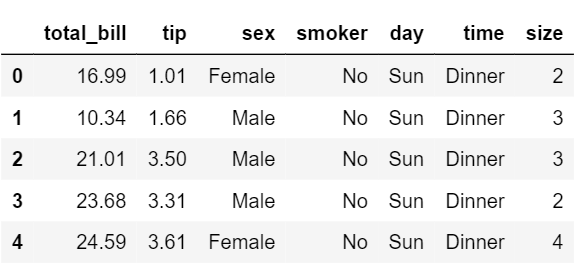Now let us begin with the regression plots in seaborn.
Regression plots in seaborn can be easily implemented with the help of the lmplot() function. lmplot() can be understood as a function that basically creates a linear model plot. lmplot() makes a very simple linear regression plot.It creates a scatter plot with a linear fit on top of it.
Simple linear plot

 `sns.set_style(``'whitegrid'``) ` `sns.lmplot(x ``=``'total_bill'``, y ``=``'tip'``, data ``=` `dataset) `

Output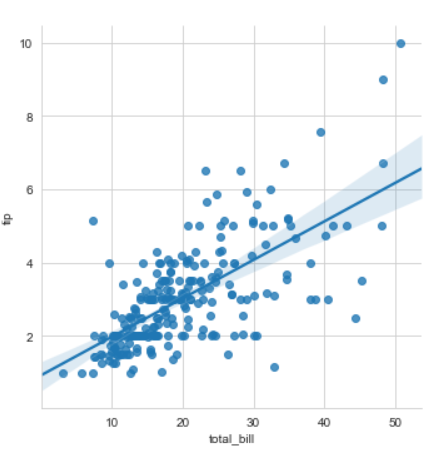Explanation
x and y parameters are specified to provide values for the x and y axes. sns.set_style() is used to have a grid in the background instead of a default white background. The data parameter is used to specify the source of information for drawing the plots.

 `sns.set_style(``'whitegrid'``) ` `sns.lmplot(x ``=``'total_bill'``, y ``=``'tip'``, data ``=` `dataset,  ` `           ``hue ``=``'sex'``, markers ``=``[``'o'``, ``'v'``]) `

Output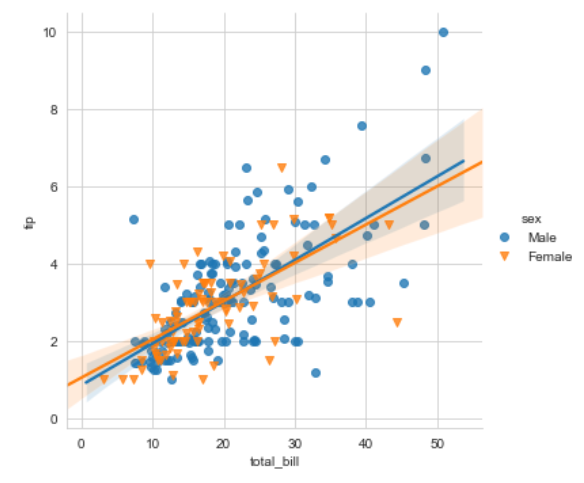Explanation
In order to have a better analysis capability using these plots, we can specify hue to have a categorical separation in our plot as well as use markers that come from the matplotlib marker symbols. Since we have two separate categories we need to pass in a list of symbols while specifying the marker.
Setting the size and color of the plot

 `sns.set_style(``'whitegrid'``) ` `sns.lmplot(x ``=``'total_bill'``, y ``=``'tip'``, data ``=` `dataset, hue ``=``'sex'``,  ` `           ``markers ``=``[``'o'``, ``'v'``], scatter_kws ``=``{``'s'``:``100``},  ` `           ``palette ``=``'plasma'``) `

Output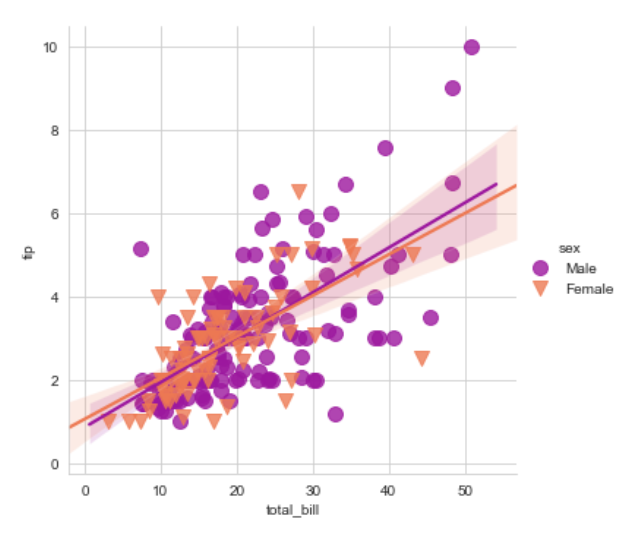Explanation
In this example what seabron is doing is that its calling the matplotlib parameters indirectly to affect the scatter plots. We specify a parameter called scatter_kws. We must note that the scatter_kws parameter changes the size of only the scatter plots and not the regression lines. The regression lines remain untouched. We also use the palette parameter to change the color of the plot.Rest of the things remain the same as explained in the first example.
Displaying multiple plots

 `sns.lmplot(x ``=``'total_bill'``, y ``=``'tip'``, data ``=` `dataset,  ` `           ``col ``=``'sex'``, row ``=``'time'``, hue ``=``'smoker'``) `

Output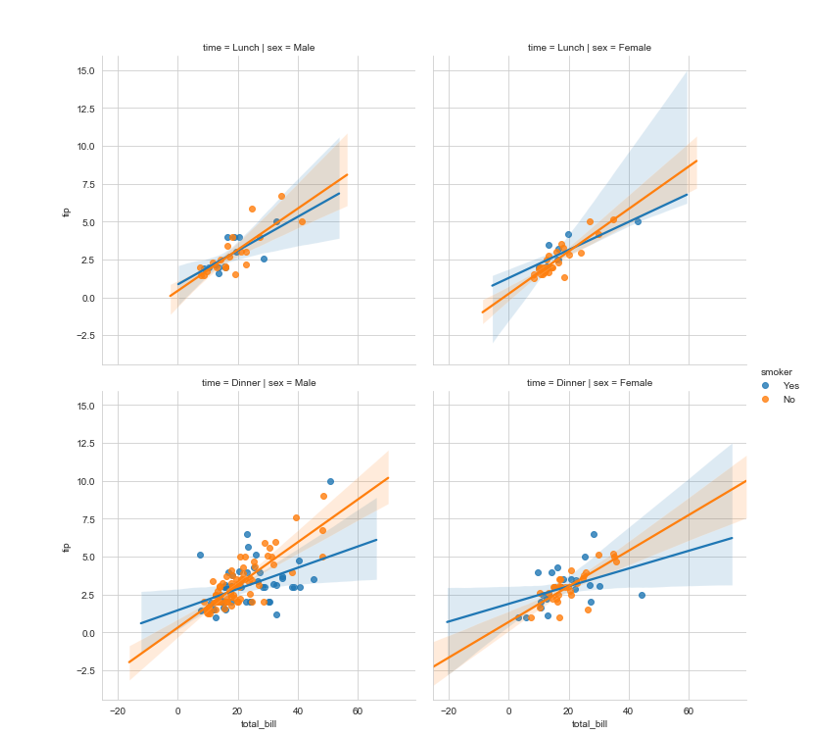Explanation
In the above code, we draw multiple plots by specifying a separation with the help of the rows and columns. Each row contains the plots of tips vs the total bill for the different times specified in the dataset. Each column contains the plots of tips vs the total bill for the different genders. A further separation is done by specifying the hue parameter on the basis of whether the person smokes.
Size and aspect ratio of the plots

 `sns.lmplot(x ``=``'total_bill'``, y ``=``'tip'``, data ``=` `dataset, col ``=``'sex'``,  ` `           ``row ``=``'time'``, hue ``=``'smoker'``, aspect ``=` `0.6``,  ` `           ``size ``=` `4``, palette ``=``'coolwarm'``) `

Output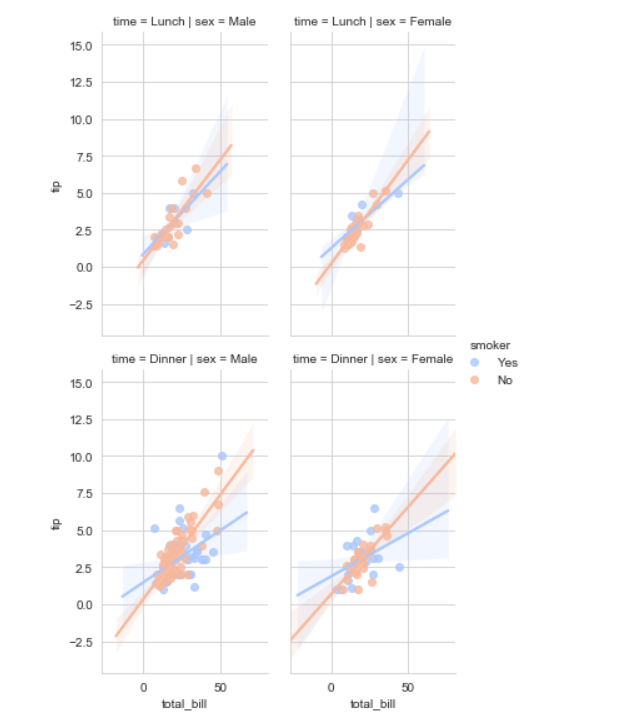Explanation
Suppose we have a large number of plots in the output, we need to set the size and aspect for it in order to better visualize it.
aspect : scalar, optional specifies the aspect ratio of each facet, so that “aspect * height” gives the width of each facet in inches.

My Personal Notes arrow_drop_upCheck out this Author's contributed articles.

If you like GeeksforGeeks and would like to contribute, you can also write an article using contribute.geeksforgeeks.org or mail your article to contribute@geeksforgeeks.org. See your article appearing on the GeeksforGeeks main page and help other Geeks.

Please Improve this article if you find anything incorrect by clicking on the "Improve Article" button below.

Article Tags :
Practice Tags :

Be the First to upvote.

Please write to us at contribute@geeksforgeeks.org to report any issue with the above content.